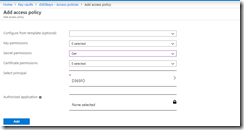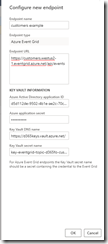Remembering Thoughts

## Charge Id – The prediction model

In this post we will build a prediction model using ML .Net to classify an individual bank transaction into a pre-defined group using the description text.

We will then evaluate the effectiveness of the model using standard evaluation metrics.

The steps involved to create a model using ML.Net are:

1. Decide what type of machine learning problem we are trying to solve?
2. Retrieve and clean the data   (CSV/TSV used with ML.Net for no)
3. Create a prediction service API
4. Create a ‘Learning Pipeline’ to iterate over the model build process
• Training
• Testing
• Evaluating result metrics
5. Exploring the output model file

## Deciding what type of machine learning problem we are trying to solve?

This cheat sheet is a good place to start to help identify the type of outcome you want from the data you have.

In this area we are using Supervised machine learning to predict a single category given an input of data [TransactionUtcDate, Description, Amount].

As we have a known set of categories (known set of labels) we will be using a multi class classifier which is described as follows:

• Multi-Class: classification task with more than two classes such that the input is to be classified into one, and only one of these classes. Example: classify a set of images of fruits into any one of these categories — apples,bananas, and oranges.

Other types available are:

• Multi-labels: classifying a sample into a set of target labels. Example: tagging a blog into one or more topics like technology, religion, politics etc. Labels are isolated and their relations are not considered important.
• Hierarchical: each category can be grouped together with similar categories, creating meta-classes, which in turn can be grouped again until we reach the root level (set containing all data). Examples include text classification& species classification.

## Retrieving and cleaning the data

Having analysed the data and problem we now have an CSV extract for a bank account statement.

We shuffle the data in the file and split this into 2 files ‘train.csv’ and ‘test.csv’ in order to perform cross validation.

## Creating a prediction service

To use our predictor we create a prediction service with 3 methods:

`````` public interface IPredict
{
}

``````

## Creating the Learning Pipeline

`````` var pipeline = new LearningPipeline
{
// load from CSV --> SubCategory manually classified), Description, Bank, Amount, AccountName, Notes, Tags
new Dictionarizer(("SubCategory", "Label")), //Converts input values (words, numbers, etc.) to index in a dictionary.
// ngram analysis over the transaction description
new TextFeaturizer("Description", "Description")
{
TextCase = TextNormalizerTransformCaseNormalizationMode.Lower,
WordFeatureExtractor = new NGramNgramExtractor(){Weighting = NgramTransformWeightingCriteria.TfIdf}
},
new TextFeaturizer("Bank", "Bank")
{
TextCase = TextNormalizerTransformCaseNormalizationMode.Lower
},
// feature column using bank and description
new ColumnConcatenator("Features", "Bank", "Description"),
//The SDCA method combines several of the best properties and capabilities of logistic regression and SVM algorithms
new StochasticDualCoordinateAscentClassifier(){Shuffle = false, NumThreads = 1},
//Transforms a predicted label column to its original values, unless it is of type bool
new PredictedLabelColumnOriginalValueConverter() {PredictedLabelColumn = "PredictedLabel"}
};

``````

Training

``````    Console.WriteLine("=============== Start training ===============");
var watch = System.Diagnostics.Stopwatch.StartNew();

_model = pipeline.Train<BankStatementLineItem, PredictedLabel>();
await _model.WriteAsync(PredictorSettings.Model1Path);

watch.Stop();
Console.WriteLine(\$"=============== End training ===============");
Console.WriteLine(\$"training took {watch.ElapsedMilliseconds} milliseconds");
Console.WriteLine("The model is saved to {0}", PredictorSettings.Model1Path);

``````

Testing

``````public async Task<string> PredictAsync(PredictionRequest request)
{
if (_model == null)
{
}

var item = new BankStatementLineItem
{
Description = request.Description,
AccountName = request.AccountName,
Amount = request.Amount,
AccountNumber = request.AccountNumber,
Bank = request.Bank,
TransactionUtcDate = request.TransactionUtcDate,
Notes = request.Notes,
Tags = request.Tags
};

var prediction = _model.Predict(item);

return prediction.SubCategory;
}
``````
##### Write an test for verify subcategories are correctly predicted from the test data
``````[Theory]
[InlineData("EFTPOS ALDI 27 CARRUM DOWNS AU","ANZ",Categories.Groceries.Supermarkets)]
[InlineData("Kmart Cannington Aus","ANZ",Categories.Shopping.OtherShopping)]
[InlineData("Bunnings Innaloo","ANZ",Categories.Home.HomeImprovement)]
[InlineData("City Of Perth Park1 Perth","ParkingTolls",Categories.Transport.ParkingTolls)]
[InlineData("Virgin Australia Airli Spring Hill Aus","WESTPAC_INTERNET_BANKING",Categories.HolidayTravel.Flights)]
[InlineData("My Healthy Place Floreat","WESTPAC_INTERNET_BANKING",Categories.HealthBeauty.Chemists)]
public async Task Predict_test_sample(string description, string bank, string subcategory)
{
var result = await _predict.PredictAsync(new PredictionRequest()
{
Description = description,
Bank =bank
});

Console.WriteLine(result);
result.Should().Be(subcategory);
}

``````

#### Evaluating result metrics

`````` public async Task<ClassificationMetrics> EvaluateAsync(string testPath)
{
var evaluator = new ClassificationEvaluator();

if (_model == null)
{
}

var metrics = evaluator.Evaluate(_model, testData);
return metrics;
}

``````

Write a test to ensure the model is giving accurate results

``````    [Fact]
{
var metrics = await _predict.EvaluateAsync(Test);
Console.WriteLine();
Console.WriteLine("PredictionModel quality metrics evaluation");
Console.WriteLine("------------------------------------------");
Console.WriteLine(\$"confusion matrix: {metrics.ConfusionMatrix}");
metrics.AccuracyMacro.Should().BeGreaterOrEqualTo(0.95);
}

``````

#### The output metrics#### Metrics for classification models

The following metrics are reported when evaluating classification models. If you compare models, they are ranked by the metric you select for evaluation.

##### AccuracyMacro

The macro-average is computed by taking the average over all the classes of the fraction of correct predictions in this class (the number of correctly predicted instances in the class, divided by the total number of instances in the class).

The macro-average metric gives the same weight to each class, no matter how many instances from that class the dataset contains.

##### AccuarcyMicro

The micro-average is the fraction of instances predicted correctly. The micro-average metric weighs each class according to the number of instances that belong to it in the dataset.

##### LogLoss

The log-loss metric, is computed as follows: _LL = - (1/m) * sum( log(p[i])) _where m is the number of instances in the test set. p[i] is the probability returned by the classifier if the instance belongs to class 1, and 1 minus the probability returned by the classifier if the instance belongs to class 0.__

##### LogLossReduction

The log-loss reduction is scaled relative to a classifier that predicts the prior for every example: (LL(prior) - LL(classifier)) / LL(prior) This metric can be interpreted as the advantage of the classifier over a random prediction. E.g., if the RIG equals 20, it can be interpreted as “the probability of a correct prediction is 20% better than random guessing”.

##### PerClassLogLoss

The log-loss metric, is computed as follows: LL = - (1/m) * sum( log(p[i])) where m is the number of instances in the test set. p[i] is the probability returned by the classifier if the instance belongs to the class, and 1 minus the probability returned by the classifier if the instance does not belong to the class.

TopKAccuracy

If OutputTopKAcc was specified on the evaluator to be k, then TopKAccuracy is the relative number of examples where the true label is one of the top k predicted labels by the predictor.

## Exploring the output model file

The model is output as a .zip file making it easy to deploy.

Extracted contents are below## Conclusion

In this post we have chosen a machine learning model to create, built a learning pipeline to ingest the data, performed training and evaluated our metrics using training data.  The end result is a deployable file alongside our application.

Next up –> hosting the model in an Azure Function app.

## Code

https://github.com/chrismckelt/vita

Published: